# MCQs on Digital Electronics

##### Page 30 of 35. Go to page 1 2 3 4 5 6 7 8 9 10 11 12 13 14 15 16 17 18 19 20 21 22 23 24 25 26 27 28 29 30 31 32 33 34 35
01․ The decimal equivalent of hexadecimal number “BEED” is
47,877
48,877
48,777
none

(BEED)16=(11×163+14×162+14×161+13×160)10 =(48,877)10

02․ Which one of the following even parity codes are in errors. (I)100110010 (II)011101010 (III)10111111010001010
Only (II)
Only (III)
Both (II)&(III)
Both (I)&(III)

No. of 1’s in (I) is 4 i.e. even parity No. of 1’s in (II) is 5 i.e. not even parity(Error) No. of 1’s in (III) is 10 i.e. even parity

03․ Which of the following shows the correct attachment of even parity bit to the data 00001001?
100001001
000010011
000001001
000010010

As option (C) has 2 ones so it is even parity and by adding zero we extend the bit size of an even parity number.

04․ If in a base b number system, 54/4=13, the base of the number is
7
6
8
9

54/4 = 13 can be written as (5b+4)/4 = b+3 5b+4 = 4b+12 b = 8

05․ Consider the operation 24+17 = 40 .What is the correct base of number so that operation is correct?
10
11
12
13

24 + 17 = 40 this representation can be written as (2b + 4) + (b+7) = (4b + 0) B = 11

06․ 9’s complement of 52784630 is
58326479
47215369
48225469
57316379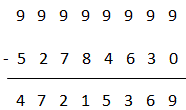07․ 10’s complement of 63325600 is
633256600
366744399
36674400
36774400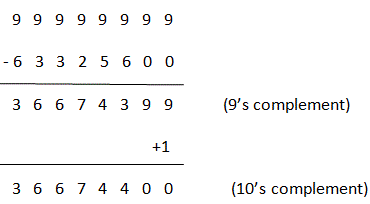08․ 16’s complement of BABA is?
4546
4445
4446
4544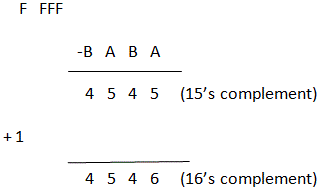09․ A self-complementing code for the decimal digits is created using the weight 4 4 3 -2. The value of 8592 in the code is
1100 1011 1111 1001
1000 0101 1001 0010
1100 1001 1111 1000
1100 0111 1111 0011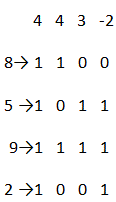Hence, (8592)10 = (1100 1011 1111 1001)443-2

10․ Which of the following BCD codes with an odd parity bit included in the end of code group, have error in the code? (I)1001010110000 (II)0100011101100 (III)0111110000011 (IV)1000011000101
Both (I) & (II)
Both (I)&(IV)
Both (II)&(III)
Only (II)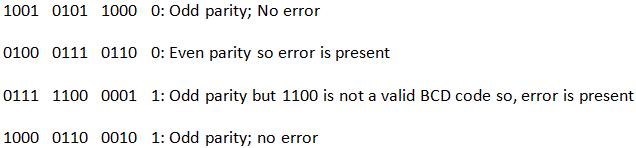<<<2829303132>>>